## Abelian Group

N.B. A detailed on-line essay by S. Finch was the starting point for this entry.

A Group for which the elements Commute (i.e.,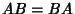for all elementsand) is called an Abelian group. All Cyclic Groups are Abelian, but an Abelian group is not necessarily Cyclic. All Subgroups of an Abelian group are Normal. In an Abelian group, each element is in a Conjugacy Class by itself, and the Character Table involves Powers of a single element known as a Generator.

No general formula is known for giving the number of nonisomorphic Finite Groups of a given Order. However, the number of nonisomorphic Abelian Finite Groupsof any given Orderis given by writingas(1)

where theare distinct Prime Factors, then(2)

whereis the Partition Function. This gives 1, 1, 1, 2, 1, 1, 1, 3, 2, ... (Sloane's A000688). The smallest orders for which, 2, 3, ... nonisomorphic Abelian groups exist are 1, 4, 8, 36, 16, 72, 32, 900, 216, 144, 64, 1800, 0, 288, 128, ... (Sloane's A046056), where 0 denotes an impossible number (i.e., not a product of partition numbers) of nonisomorphic Abelian, groups. The missing'' values are 13, 17, 19, 23, 26, 29, 31, 34, 37, 38, 39, 41, 43, 46, ... (Sloane's A046064). The incrementally largest numbers of Abelian groups as a function of order are 1, 2, 3, 5, 7, 11, 15, 22, 30, 42, 56, 77, 101, ... (Sloane's A046054), which occur for orders 1, 4, 8, 16, 32, 64, 128, 256, 512, 1024, 2048, 4096, 8192, ... (Sloane's A046055).

The Kronecker Decomposition Theorem states that every Finite Abelian group can be written as a Direct Product of Cyclic Groups of Prime Power Orders. If the Orders of a Finite Group is a Prime, then there exists a single Abelian group of order(denoted) and no non-Abelian groups. If the Order is a prime squared, then there are two Abelian groups (denotedand. If the Order is a prime cubed, then there are three Abelian groups (denoted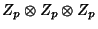,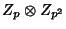, and), and five groups total. If the Order is a Product of two primesand, then there exists exactly one Abelian group of order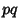(denoted).

Another interesting result is that ifdenotes the number of nonisomorphic Abelian groups of Order, then(3)

whereis the Riemann Zeta Function. Srinivasan (1973) has also shown that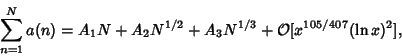(4)

where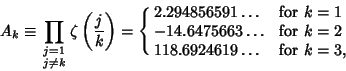(5)

and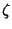is again the Riemann Zeta Function. [Richert (1952) incorrectly gave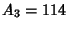.] DeKoninck and Ivic (1980) showed that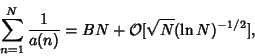(6)

where(7)

is a product over Primes. Bounds for the number of nonisomorphic non-Abelian groups are given by Neumann (1969) and Pyber (1993).

See also Finite Group, Group Theory, Kronecker Decomposition Theorem, Partition Function P, Ring

References

DeKoninck, J.-M. and Ivic, A. Topics in Arithmetical Functions: Asymptotic Formulae for Sums of Reciprocals of Arithmetical Functions and Related Fields. Amsterdam, Netherlands: North-Holland, 1980.

Erdös, P. and Szekeres, G. Über die Anzahl abelscher Gruppen gegebener Ordnung und über ein verwandtes zahlentheoretisches Problem.'' Acta Sci. Math. (Szeged) 7, 95-102, 1935.

Finch, S. Favorite Mathematical Constants.'' http://www.mathsoft.com/asolve/constant/abel/abel.html

Kendall, D. G. and Rankin, R. A. On the Number of Abelian Groups of a Given Order.'' Quart. J. Oxford 18, 197-208, 1947.

Kolesnik, G. On the Number of Abelian Groups of a Given Order.'' J. Reine Angew. Math. 329, 164-175, 1981.

Neumann, P. M. An Enumeration Theorem for Finite Groups.'' Quart. J. Math. Ser. 2 20, 395-401, 1969.

Pyber, L. Enumerating Finite Groups of Given Order.'' Ann. Math. 137, 203-220, 1993.

Richert, H.-E. Über die Anzahl abelscher Gruppen gegebener Ordnung I.'' Math. Zeitschr. 56, 21-32, 1952.

Sloane, N. J. A. Sequence A000688/M0064 in An On-Line Version of the Encyclopedia of Integer Sequences.'' http://www.research.att.com/~njas/sequences/eisonline.html and Sloane, N. J. A. and Plouffe, S. The Encyclopedia of Integer Sequences. San Diego: Academic Press, 1995.

Srinivasan, B. R. On the Number of Abelian Groups of a Given Order.'' Acta Arith. 23, 195-205, 1973.